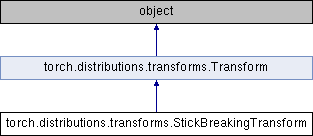Caffe2 - Python API A deep learning, cross platform ML framework
torch.distributions.transforms.StickBreakingTransform Class Reference
Inheritance diagram for torch.distributions.transforms.StickBreakingTransform:## Public Member Functions

def __eq__ (self, other)

def log_abs_det_jacobian (self, x, y)Public Member Functions inherited from torch.distributions.transforms.Transform
def __init__ (self, cache_size=0)

def inv (self)

def sign (self)

def __eq__ (self, other)

def __ne__ (self, other)

def __call__ (self, x)

def log_abs_det_jacobian (self, x, y)

def __repr__ (self)

## Static Public Attributes

domain

codomain

bijective

event_dimStatic Public Attributes inherited from torch.distributions.transforms.Transform
bijective

event_dim

## Detailed Description

```Transform from unconstrained space to the simplex of one additional
dimension via a stick-breaking process.

This transform arises as an iterated sigmoid transform in a stick-breaking
construction of the `Dirichlet` distribution: the first logit is
transformed via sigmoid to the first probability and the probability of
everything else, and then the process recurses.

This is bijective and appropriate for use in HMC; however it mixes
coordinates together and is less appropriate for optimization.
```

Definition at line 473 of file transforms.py.

The documentation for this class was generated from the following file: